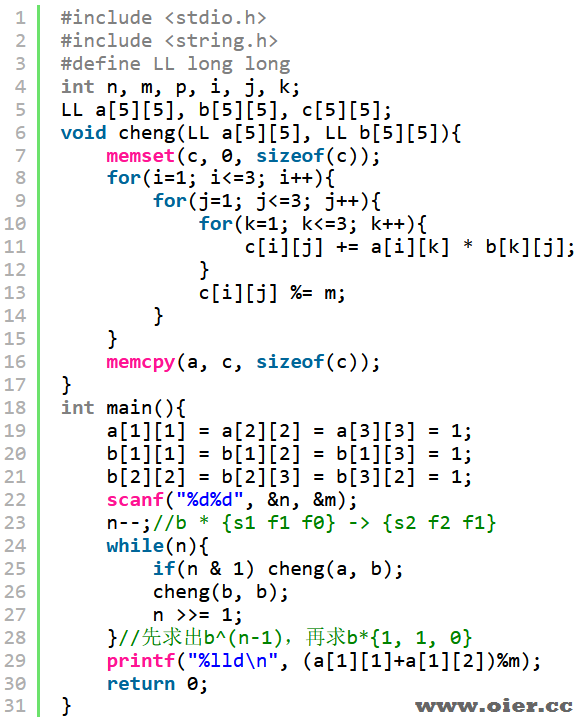SSOJ2923Fibonacci前n项和
126+

## 提示

#### 样例输入

5 1000

#### 样例输出

12

## 程序实现$s_n = s_{n-1} + f_n$，$f_n = f_{n-1} + f_{n-2}$

$s_n$

$f_{n}$

$f_{n-1}$

$s_{n+1}$

$f_{n+1}$

$f_{n}$

1 1 1

0 1 1

0 1 0

1 0 0

0 1 0

0 0 1### About crxis

SSOJ2923Fibonacci前n项和：等您坐沙发呢！